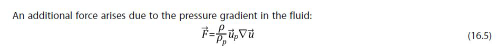# 拉格朗日梯度项方程

• 在拉格朗日粒子控制方程中，有压力梯度项：
\begin{equation}
\mathrm{Force}=\frac{\mathrm{mass}}{\rho}\nabla p
\end{equation}
但是OpenFOAM中植入的方程为：
\begin{equation}
\mathrm{Force}=\mathrm{mass}\frac{\rho_\mathrm{c}}{\rho_\mathrm{d}}\frac{\mathrm{D} \mathbf{U}}{\mathrm{D} t}
\end{equation}
有人知道这个方程怎么来的么？

•ANSYS Fluent里面这么计算的。压力梯度项和压力无关？

• @李东岳 把压力梯度视为流体产生加速度的原因，压力梯度力=质量*加速度，我在二相欧拉法里面看过类似的做法，拉格朗日方程不太懂

• 好吧，你说的很对，看来就是：
\begin{equation}
\frac{\nabla p}{\rho}=\frac{\mathrm{D} \mathbf{U}}{\mathrm{D} t}
\end{equation}